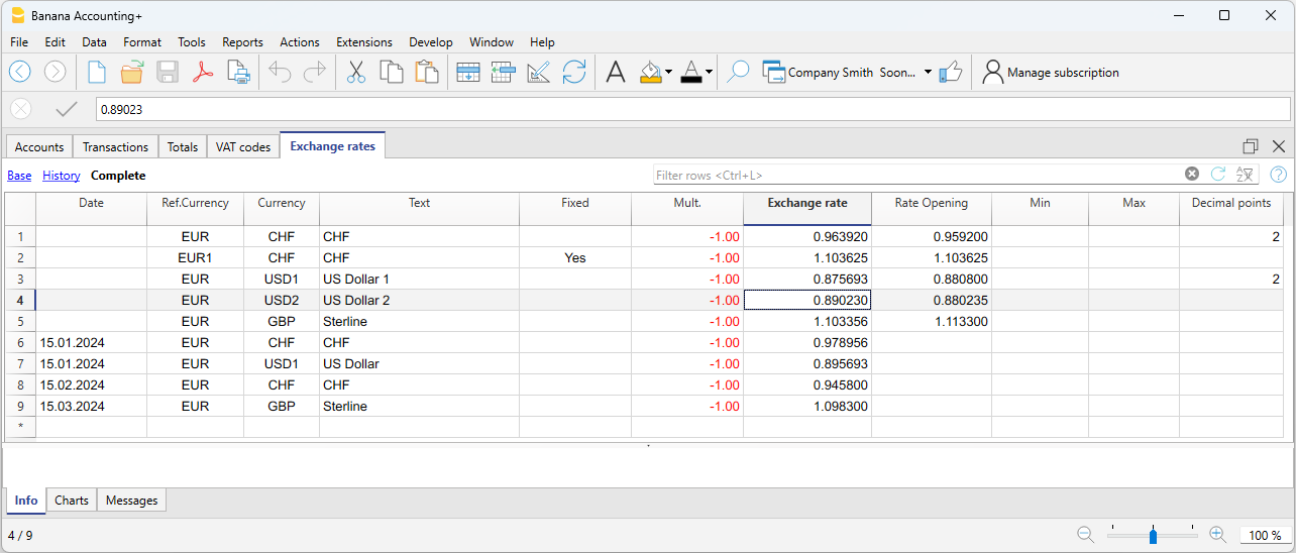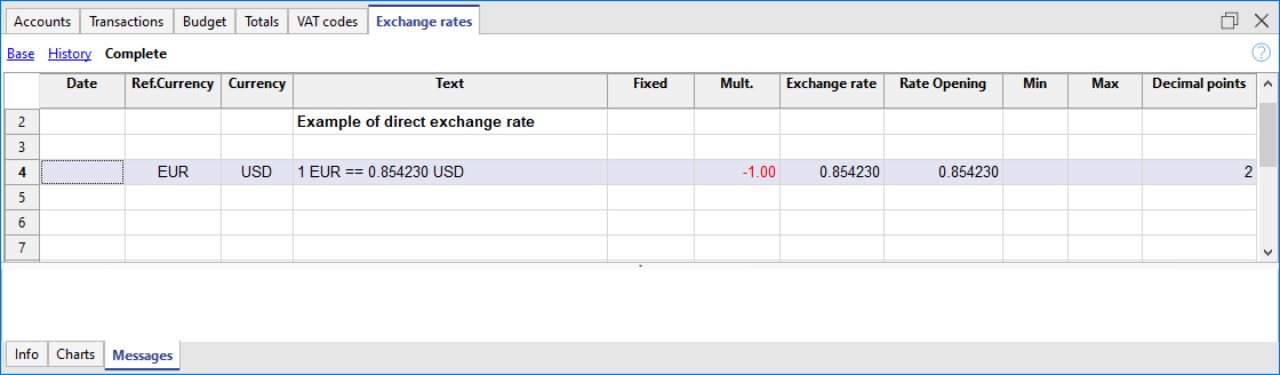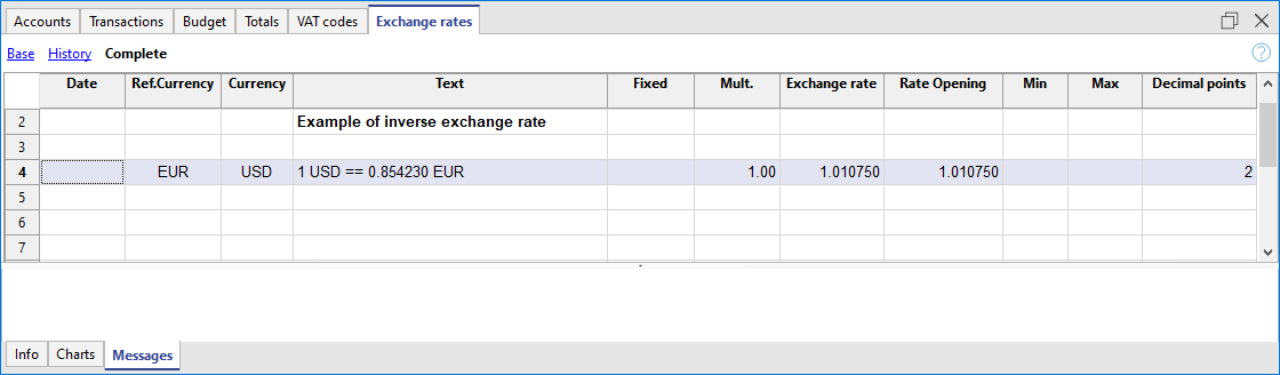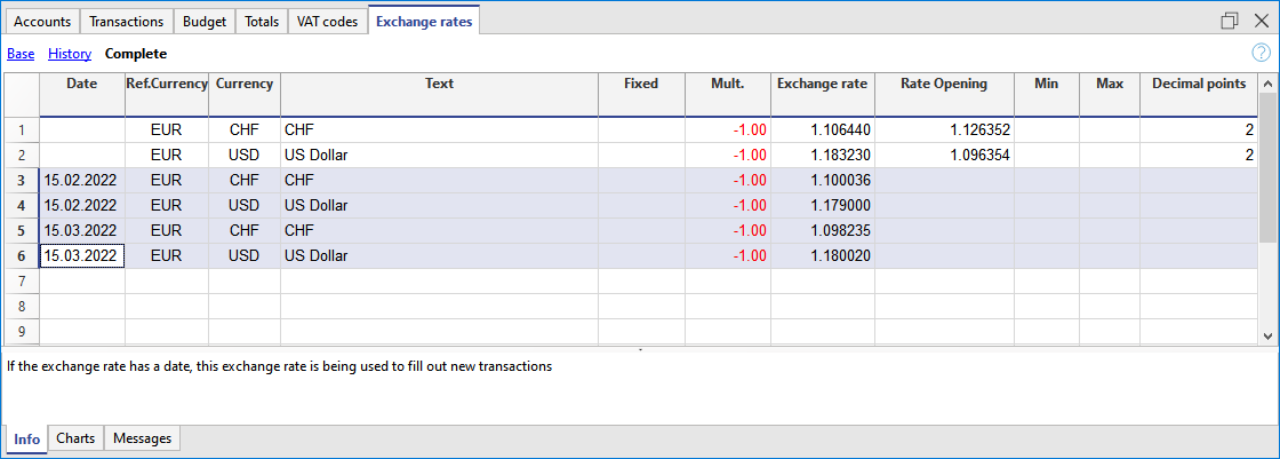# Exchange rates table

Documentation •

In the Exchange rates table you can define any currency and its current and opening exchange rate.

Before entering multi-currency transactions it is necessary to define the parameters of the used currencies in the Exchange rates table.## Rows with and without date

There are two types of rows for the exchange rate:

1. Without date, Reference exchange rates for the program.
They are used by the program for the most recurrent conversion operations.
For each foreign currency used there must be an exchange row without the date.
The meaning is therefore the following:
• Exchange rate column
It is the exchange rate used by the program as current exchange rate or respectively as closing exchange rate.
• Current exchange rate.
• It is the exchange rate proposed automatically in the transactions, when there is no historical exchange (exchange rows with date).
If you modify the exchange rate in the Exchange rates table, the exchange rates and amounts used in the journal rows are not changed.
• It is used for the calculation of the balance column calculated in the accounts table and for the conversion to Currency 2.
• Closing exchange rate.
• Opening exchange rate column
• Is used In the Accounts table to convert the opening amount of the foreign currency into the opening amount of the basic accounting currency.
• If the opening exchange rate is changed, the accounting must be recalculated.
2. With date (historical exchange rates)
In the cases indicated here, the program chooses the exchange rate with a date equal to or less than the date of the transaction row.

• When entering transactions, if there is a historical exchange rate, this is proposed and used as the default exchange rate.
• When creating exchange rate differences and specifying to use historical exchange rate.
• In rows with a date, the opening exchange rate is not used.

## Columns of the Exchange rate table

All columns are displayed in the Base view.

#### Date

• Without date. Reference exchange rate row used for the program.
• With date. Historical exchange rate.

### Ref. Currency

It is the starting currency for the exchange (EUR in the example).

### Currency

This is the destination currency, into which the value of the “Ref. Currency” will be converted.

### Text

A text to indicate the foreign currency that we are dealing with.

### Fixed

True or false. If there is a fixed exchange rate, enter Yes in this column. The used exchange rate is specified in the Exchange Rate column.

### Mult. (Multiplier)

The multiplier is usually 1, 100 or 1000 and is used to obtain the effective exchange rate. The multiplier is used for currencies which have a very low unit value in order to avoid having to insert exchange rates with many zeros.

In our models, the negative multiplication (-1) is used in the Multiplier column of the Exchange rates table.
In this case:

• In the Ref. (Reference) Currency column, the base currency of the accounting system is entered
•  In the Currency column, the foreign currency is entered.

When in the Ref. Currency and Currency columns the abbreviations of the currencies are reversed, the Multiplier 1 (positive exchange rate) must be entered in the column.

If transactions have already been made with the same currency, do not change the multiplier, otherwise the programme will report errors in the entries due to incorrect exchange rates.

### Exchange rate

The current or closing exchange rate of the currency against the reference currency.
It is also used to calculate exchange rate differences. Before calculating the exchange rate differences or closing the accounting, it is necessary to update the value by inserting the closing exchange rate.

The exchange rate and the multiplier are being applied according to the following formulas

• With multiplier > 0
Currency amount = Ref. currency amount* (exchange rate / |mult.|)

• With multiplier < 0
Ref. currency amount = Currency amount * (exchange rate / |mult.|)

### Opening Exchange rate

This is the exchange rate at the moment the accounting is opened. To be indicated only on a row without date.

• It is used to convert the opening amount of the foreign currency into the opening amount of the accounting’s basic currency.
• Should be corresponding to the closing exchange rate of the preceding year.
If the closing exchange rates are not equal to the opening exchange rates, the Assets and Liabilities might result into different totals; see Differences in the Opening Balances.
• The opening exchange rate should never be changed in the course of the year, otherwise exchange rate differences are being created in the total of the opening balances.
• When creating a new year or when updating the opening balances, the program defines the opening exchange rate with the value indicated in the Exchange rate column (row without date) of the accounting of the previous year.

### Minimum

The minimum exchange rate accepted. If an inferior exchange rate is used for the transactions, there will be a warning.

### Maximum

This column shows the maximum exchange rate accepted. If a superior exchange rate is used for the transactions, there will be a warning.

### Decimal Points

The number of decimal points to be used when rounding the amounts of the currency.

## Modifications in the Exchange rate table

The exchange rates entered in the transactions are standalone and independent of the exchange rates shown in the Exchange rate table. If you change an exchange rate in the Exchange rate table, there will be no repercussions in the transactions already submitted.

However, the following modifications do have repercussions:
• Modification of the Opening exchange rate
The next time you recalculate the accounting, the balances in basic currency of the accounts will be recalculated with the new exchange rate. Therefore, pay attention when modifying the Opening exchange rates once you have inserted the opening balances.
If you modify the Opening exchange rates and there are opening balances, it is important to recalculate the accounting.
• Modification of the multiplier
• When changing the multiplier of a currency already used in the Transactions table, the program will report a warning as soon as the accounting is being recalculated or will move to the transaction row.
The transaction amount and the correct amount in the basic currency will have to be reentered.
• When the accounting is being recalculated, the opening balances in basic currency will be recalculated as a result.

## Direct exchange rates

Direct exchange rates are those where the basic currency and the foreign currency are indicated on the same row.
In the example below, the direct exchange rate is EUR → USD.## Indirect exchange rates

Indirect exchange rates are those where a direct exchange between two currencies is not being indicated (not recommended).
The exchange rate is deducted by the program based on other combinations of entered exchange rates

In the example, the EUR → USD exchange rate has not been defined, and the program deduces it by combining the EUR → USD and USD → EUR exchange rates.

The program supports indirect exchange rates. It is advisable to use only direct exchange rates and avoid using accounts where the direct exchange rate between the base currency and the account currency is not specified. With indirect exchange rates it is might be possible that it will not not always be clear which exchange rate is being applied.## Historical exchange rates

As explained above, if an exchange has a date, the program considers it to be a historical exchange rate.
In the cases indicated here, the program chooses the exchange rate with a date equal to or less than the date of the transaction row.

• When entering transactions, if there is a historical exchange rate, it is proposed and used as the default exchange rate.
• When the exchange rate differences transactions are created and the option to use the historical exchange rate is activated.
• In rows with a date, the opening exchange is not used.You can import exchange rates from another .ac2 file or copy / paste historical exchange rates from Excel for example.
If you import several historical exchange rates at the end of your accounting period, that is, when you have already entered accounting transactions, simply manually delete the transactions exchange rates (Exchange rate column, Transactions table) to make sure that the program uses the imported exchange rates instead of those entered in the transactions.

## Monthly average exchange rate

If it is necessary to have a monthly average exchange rate, this must be inserted manually in the Exchange rate table, in a row without date. In the Transactions table, for foreign currency accounts, the average exchange rate will always be included until it is updated again in the Exchange rate table.

For the monthly average exchange rate, we recommend consulting the page of the exchange rates proposed by the Swiss Confederation, available in Italian, French or German.

help_id
multicurrency::exchangerates